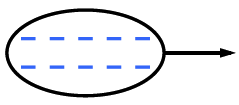### Home > MC1 > Chapter 6 > Lesson 6.2.3 > Problem6-74

6-74.

Simplify each of the following expressions.

1.  $−8 + \left(−8\right) + \left(−8\right) + 24$

$0$

1.  $−10 − \left(−10\right)$

Use the diagram below to help you visualize subtracting $−10$.1.  $36 + \left(−6\right) · 5$

$6$

1.  $10\left(2\right) − \left(−2\right)\left(10\right)$

$20 − \left(−20\right)$

1.  $3 + \left(−2\right)\left(3\right) − 3$

$−6$

1.  $98 + \left(−2\right)\left(10\right) − 9\left(5\right)$

Circling terms should be helpful with this one.

$\enclose{circle}[mathcolor="orange"]{\color{black}{98}} + \enclose{circle}[mathcolor="orange"]{\color{black}{-2(10)}}- \enclose{circle}[mathcolor="orange"]{\color{black}{9(5)}}$